Test: Constructions - 1

# Test: Constructions - 1

Test Description

## 25 Questions MCQ Test NCERT Mathematics for CAT Preparation | Test: Constructions - 1

Test: Constructions - 1 for Class 10 2022 is part of NCERT Mathematics for CAT Preparation preparation. The Test: Constructions - 1 questions and answers have been prepared according to the Class 10 exam syllabus.The Test: Constructions - 1 MCQs are made for Class 10 2022 Exam. Find important definitions, questions, notes, meanings, examples, exercises, MCQs and online tests for Test: Constructions - 1 below.
Solutions of Test: Constructions - 1 questions in English are available as part of our NCERT Mathematics for CAT Preparation for Class 10 & Test: Constructions - 1 solutions in Hindi for NCERT Mathematics for CAT Preparation course. Download more important topics, notes, lectures and mock test series for Class 10 Exam by signing up for free. Attempt Test: Constructions - 1 | 25 questions in 25 minutes | Mock test for Class 10 preparation | Free important questions MCQ to study NCERT Mathematics for CAT Preparation for Class 10 Exam | Download free PDF with solutions
 1 Crore+ students have signed up on EduRev. Have you?
Test: Constructions - 1 - Question 1

### To divide a line segment AB in the ratio 5 : 7, first a ray AX is drawn so that ∠BAX is an acute angle and then at equal distances points are marked on the ray AX such that the minimum number of these points is :

Detailed Solution for Test: Constructions - 1 - Question 1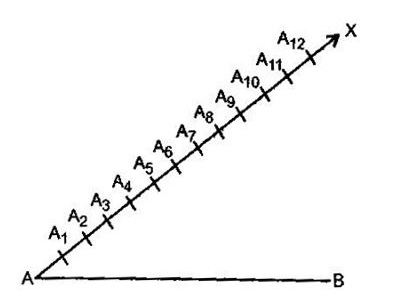According to the question, the minimum number of those points which are to be marked should be (Numerator + Denominator) i.e., 5 + 7 = 12

Test: Constructions - 1 - Question 2

### To divide a line segment AB in the ratio p : q ( p, q are positive integers), draw a ray AX so that ∠BAX s an acute angle and then mark points on ray AX at equal distances such that the minimum number of these points is :

Detailed Solution for Test: Constructions - 1 - Question 2

According to the question, the minimum number of those points which are to be marked should be (Numerator + Denominator) i.e., p + q

Test: Constructions - 1 - Question 3

### To construct a triangle similar to given ΔABC with its sides 8585 of the corresponding sides of ΔABC, draw a ray BX such that ∠CBX is an acute angle and X is one the opposite side of A with respect to BC. The minimum number of points to be located at equal distances on ray BX is :

Detailed Solution for Test: Constructions - 1 - Question 3

According to the question, the minimum number of points to be located at equal distances on ray BX is 8.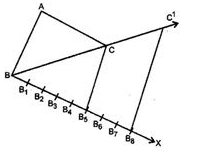Test: Constructions - 1 - Question 4

To divide a line segment AB in the ratio 5 : 6 draw a ray AX such that ∠BAX is an acute angel, then draw a ray BY parallel to AX and the points A_(1 ,) A_(2 ,) A_(3 ,) … and B_(1 ,) B_(2 ,) B_(3 ,)… are located a equal distances on ray AX and BY, respectively, Then the points joined are :

Detailed Solution for Test: Constructions - 1 - Question 4

The ratio is 5:6
Therefore, total number of divisons on BY and AX is 5+6=11.
ΔAOX and BOY are similar, since AX∥BY
⇒ ∠X = Y and ∠A = B
no. of divisions on B/no. of divisions on A = 6/5
number of divisions on B
⇒ number of divisions on B = 6/5× number of divisions in A
⇒ 6/5× 5 = 6
So, A5 should be joined to B6.

Test: Constructions - 1 - Question 5

To construct a triangle similar to given ΔABC with its sides 3/7 of the corresponding sides of ΔABC, first draw a ray BX such that ∠CBXis an acute angle and X lies on the opposite side of A with respect to BC. Then locate points B1,B2,B3, on BX equal distance and next step is to join :

Detailed Solution for Test: Constructions - 1 - Question 5

According to the question, the next step is to join is B7 to C.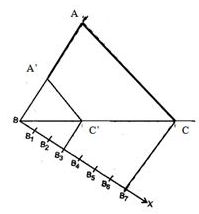Test: Constructions - 1 - Question 6

To divide a line segment AB in the ration 4 : 7, a ray AX is drawn first such that ∠BAX is an acute angle and then points A1,A2,A3,…are located at equal distances on the ray AX and the point B is joined to :

Detailed Solution for Test: Constructions - 1 - Question 6

According to question, the point B is joined to A11.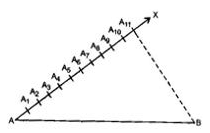Test: Constructions - 1 - Question 7

To divide a line segment AB in the ration 2 : 5, first a ray AX is drawn, so that ∠BAX is an acute angle and then at equal distances points are marked on the ray such that the minimum number of these points is :

Detailed Solution for Test: Constructions - 1 - Question 7

According to the question, the minimum number of those points which are to be marked should be (Numerator + Denominator) i.e. , 2 + 5 = 7

Test: Constructions - 1 - Question 8

To construct a triangle similar to given ΔABC with its sides 3/7 of the corresponding sides of ΔABC draw a ray BX such that ΔCBX is an acute angle and X is on the opposite side of A with respect to BC. The minimum number of points to be located at equal distances on ray BX is :

Detailed Solution for Test: Constructions - 1 - Question 8

According to question, the minimum number of points to be located at equal distances on ray BX is 7.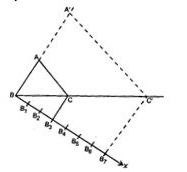Test: Constructions - 1 - Question 9

To divide a line segment PQ in the ratio 2 : 7, first a ray PZ is drawn so that ∠QPX is an acute angle and then at equal distances points are marked on the ray PX such that the minimum number of these points is :

Detailed Solution for Test: Constructions - 1 - Question 9

According to the question, the minimum number of those points which are to be marked should be (Numerator + Denominator) i.e., 2 + 7 = 9

Test: Constructions - 1 - Question 10

To divide a line segment LM in the ratio a : b, where a and b are positive integers, draw a ray LX so that ∠MLX is an acute angle and then mark points on the ray LX at equal distances such that the minimum number of these points is :

Detailed Solution for Test: Constructions - 1 - Question 10

According to the question, the minimum number of those points which are to be marked should be (Numerator + Denominator) i.e., a + b

Test: Constructions - 1 - Question 11

To construct a triangle similar to given ΔABC with its sides 7/4 of the corresponding sides of ΔABC, draw a ray BX such that ∠CBX is an acute angle and X is on the opposite side of A with respect to BC. The minimum number of points to be located at equal distances on ray BX is :

Detailed Solution for Test: Constructions - 1 - Question 11

When numerator is greater than denominator, then number of arcs should be drawnlarger of m and n. Therefore, according to question, the minimum number of points to be located at equal distances on ray BX is 7.

Test: Constructions - 1 - Question 12

To divide a line segment LM in the ratio 4 : 3, a ray LX is drawn firs such that ∠MLX is an acute angle and then points L1, L2, L3, … are located at equal distances on the ray LX and the points M is joined to :

Detailed Solution for Test: Constructions - 1 - Question 12

To divide a line segment AB in the ratio m : n, a ray AX is drawn in such that ∠BAX is an acute angle and then points A1, A2, A3, …….., Am, …….. An are located at equal distances on the ray AX and then the point B is joined to AnB.
• Therefore, according to question, the point M is joined to L7M.

Test: Constructions - 1 - Question 13

To construct a triangle similar to given ΔPQR with its sides 5/8 of the corresponding sides of ΔPQR, first a ray PXis drawn such that ∠QPX is an acute angle and X lies on the opposite side of R with respect to PQ. Then locate points P1, P2, P3…. OnPX at equal distances and next step is to join :

Detailed Solution for Test: Constructions - 1 - Question 13

To construct a triangle similar to given ΔABC with its sides mnmn of the corresponding sides of ΔABC, first a ray AX is drawn such that ∠BAX is an acute angle and X lies on the opposite side of C with respect to AB. Then locate points A1, A2, A3…. Am ….. An on AX at equal distances and next step is to join AnB. Therefore, according to question, the next step is to join P8 to Q.

Test: Constructions - 1 - Question 14

To divide a line segment AB in the ratio 3 : 7 , draw a ray AX such that ∠BAX is an acute angle, then draw a ray BY parallel to AX and the points A1,A2,A3, … and B1,B2,B3,… are located at equal distances on ray AX and BY respectively. Then the points joined are :

Detailed Solution for Test: Constructions - 1 - Question 14

If to divide the line segment AB in the ratio m : n, then we draw a ray AX such that ∠BAX is an acute angle, then draw a ray BY parallel to AX and the points A1,A2,A3, …….. Am-1, Am and B1,B2,B3, ….., Bn are located at equal distances on ray AX and BY respectively. Then the points joined are Am and Bn. Therefore, according to the question, the points joined are A3andB7.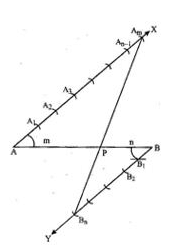Test: Constructions - 1 - Question 15

A divides the line segment PQ in the ratio :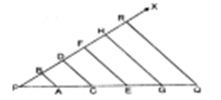Detailed Solution for Test: Constructions - 1 - Question 15

In the given figure, A divides the line segment PQ in the ratio 1 : 4.

Test: Constructions - 1 - Question 16

To draw a pair of tangents to a circle which are inclined to each other at an angle of 35°, it is required to draw tangents at the end-points of those two radii of the circle, the angle between which is

Test: Constructions - 1 - Question 17

To divide a line segment PQ in the ratio 5 : 7, first a ray PX is drawn so that ∠QPX is an acute angle and then at equal distances points are marked on the ray PX such that the minimum number of these points is

Test: Constructions - 1 - Question 18

The ____________ is the term used to denote a method in which case is driven into the ground and the material inside the casing is washed out and brought to the surface for inspection.

Detailed Solution for Test: Constructions - 1 - Question 18

The process of wash boring consists in driving an inner tube of diameter 25 mm to 50 mm, inside an outer tube of diameter 100 mm to 150 mm. Whereas, percussion boring and rotary drilling are the types of Deep boring.

Test: Constructions - 1 - Question 19

To divide a line segment AB internally in the 5 : 2, first a ray AX is drawn so that ∠BAX is an acute angle and then points A1,A2,A3.... are located at equal distance on ray AX and point B is joined to

Detailed Solution for Test: Constructions - 1 - Question 19

To divide a line segment AB in the ratio m : n (when m > n), a ray AX is drawn in such that ∠BAX is an acute angle and then points A1,A2,A3...Am,…..An…. are located at equal distances on the ray AX and then the point B is joined to AnB.
Therefore, according to the question, point B is joined toA7 .

Test: Constructions - 1 - Question 20

To divide a line segment AB internally in the ratio 4 : 7 , first a ray AX is drawn so that ∠BAX is an acute angle and then at equal distances points are marked on ray AX such that the minimum number of these points is

Detailed Solution for Test: Constructions - 1 - Question 20

According to the question, the minimum number of those points which are to be marked should be (Numerator + Denominator) i.e., 4 + 7 = 11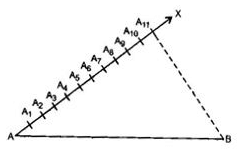Test: Constructions - 1 - Question 21

If the construction of a triangle ABC in which AB = 6 cm, ∠A = 70° and ∠B = 40° is possible then find the measure of ∠C.

Test: Constructions - 1 - Question 22

To construct a triangle similar to a given triangle ABC with its sides 2/3 of the corresponding sides side of A with respect to BC. Then locate points X1,X2,X3… at equal distance on BX. The points to be joined in the next step are

Detailed Solution for Test: Constructions - 1 - Question 22

The points to be joined in the next step are Xand C.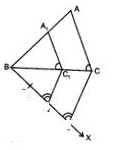Test: Constructions - 1 - Question 23

To construct a triangle similar to given ΔABC with its sides 7/5 of the corresponding side of ΔABC,draw a ray BX such that ∠CBX is an acute angle and X is on the opposite side of A with respect to BC. Then, locate points X1,X2,X3… at equal distances on BX. The points to be joined in the next step are

Detailed Solution for Test: Constructions - 1 - Question 23

According to the question, the points to be joined are Xand C.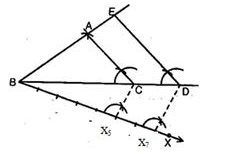Test: Constructions - 1 - Question 24

To divide line segment AB in the ration m : n (m, n are positive integers), draw a ray AX so that ∠BAX is an acute angle and then mark points on ray AX at equal distances such that the minimum number of these points is

Detailed Solution for Test: Constructions - 1 - Question 24

To divide line segment AB in the ration m : n (m, n are positive integers), draw a ray AX so that ∠BAX is an acute angle and then mark points on ray AX at equal distances such that the minimum number of these points is m + n.

Test: Constructions - 1 - Question 25

To divide a line segment AB in the ratio 5 :7, first a ray AX is drawn so that ∠BAX is an acute angle and then at equal distances, points are marked on the ray AX such that the minimum number of these points is :

Detailed Solution for Test: Constructions - 1 - Question 25

According to the question, the minimum number of those points which are to be marked should be (Numerator + Denominator) i.e., 5 + 7 = 12

## NCERT Mathematics for CAT Preparation

276 docs|149 tests
 Use Code STAYHOME200 and get INR 200 additional OFF Use Coupon Code
Information about Test: Constructions - 1 Page
In this test you can find the Exam questions for Test: Constructions - 1 solved & explained in the simplest way possible. Besides giving Questions and answers for Test: Constructions - 1, EduRev gives you an ample number of Online tests for practice

## NCERT Mathematics for CAT Preparation

276 docs|149 tests